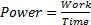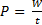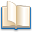# Electromotive Force and Potential difference

## Electromotive Force and Potential difference

The following text is used only for teaching, research, scholarship, educational use and informative purpose following the fair use principles.

We thank the authors of the texts and the source web site that give us the opportunity to share their knowledge

# W = VQ

Electrical Power, Potential difference and Current
We have seen in chapter 11 that
Power is defined as the rate at which work is done or
Power is the rate at which energy is converted from one form to another.
Most energy conversions in this section involve electrical energy converting to heat energy.Remember 1 watt = 1 joule per second

Now if we take W = VQ and divide both sides by time (t), we get P = VI (because W/t = P, and Q/t = I)

P = VI

Measuring Potential Difference

• Voltages in Series:      VTotal = V1 + V2
• Voltages in parallel are the same.*
• A Voltmeter is used to measure Potential Difference.
• A Voltmeter is always connected in parallel with whatever it is measuring.

Electromotive Force (emf)

A Voltage when applied to a full circuit is called an emf*.
The unit of emf is also the Volt

Some Sources of Electromotive Force (emf)

• Mains
• Simple Cell
• Dry batteries
• Thermocouple

Leaving Cert Physics Syllabus

 Content Depth of Treatment Activities STS Sources of emf and electric current Pd and voltage are the same thing; they are measured in volts. A voltage when applied to a circuit is called an emf. Sources of emf: mains, simple cells, lead-acid accumulator, car batteries, dry batteries, thermocouple.

Extra Credit
We have noted already that one way of defining potential difference is by saying it is the work done when bringing a charge from one point to another.
This suggests that something has to do work on the charge.
But this is only if the charge in question is similar in sign to the sign of the charge it is going to, e.g. electron to negative terminal, or proton to positive terminal.
Alternatively we could define potential difference as the work done by the charge in going from one place to another, e.g. an electron moving towards a positive terminal.

But what does it mean to say that ‘the charge is doing work’?
Well, work is a form of energy, so the charge must be converting energy from one form to another (anytime work is being done, energy is being converted from one form to another).
In this case potential energy is being converted to kinetic energy (the charge speeds up), and when the charge reaches the terminal this kinetic energy gets converted in to a combination of heat and/or chemical energy.

Bottom Line, remember W = VQ?
This can be rearranged to give V = W/Q, which in physical terms means that the potential difference between two points can be defined as the work done as the charge goes from one point to the othe,r divided by the charge itself.

Don’t be too surprised if you find this stuff confusing. Not only is it difficult to understand an abstract concept (it’s not like you can hold a bunch of voltage in your hand), but the meaning of the term ‘voltage’ has itself changed over the years, and you’re left to make sense of the remaining muddle!
If you think I’m just saying this to make you feel better, then read on.

The following extract has been taken from the minutes of a History of Science meeting, in 2002.
John Roche, of Linacre College, Oxford, opened the session after tea, speaking on the concept of voltage. He began by claiming that almost every concept in electricity and electromagnetism is ambiguous, and the concept of voltage is one of the most incoherent. Its evolution in difficult to follow.
Abbé Nollet, in the 18th century, distinguished quantity and degree of electrification. Others made similar distinctions between quantity and intensity or tension or pressure – what we would call voltage.
Roche showed how the term “voltage” had come to be used nowadays in three different ways; for electromotive force, potential difference and (absolute) potential.
Volta defined electrical tension as the endeavour of the electrical fluid to escape from a body. Volta’s tension was more akin to a force, unlike the modern definition of electromotive force, which is a misnomer, being defined in terms of energy.
Ohm carried Volta’s concept to closed circuits with the idea that voltage was proportional to the difference in tension between the ends of a conductor. For Ohm, it was the gradient of electrical tension that drove the current.
Poisson introduced an entirely different concept, of charge divided by distance to a point, which Green called the potential. This was an analytical device only, arising from an analogy with Laplace’s gravitational potential function.
Kirchhoff reconciled Volta’s tension with Poisson’s potential function through the concept of energy or vis viva introduced by Helmholtz. From Kirchhoff, current is driven by the electric field in a conductor and voltage is related to the energy supplied, but physicists and electrical engineers do not usually think of them in this way.
All the earlier interpretations remain current, but with different weights, and most of the time voltage is seen as a driving energy.
IOP History of Physics Group Newsletter, Spring 2000, page 65

*Voltages in parallel are the same
This is a constant source of confusion for students and indeed I suspect for many teachers.
Allow me to try and clarify.
Consider two resistors in parallel: R1 is 100 Ohms and is in parallel with R2 - a 20 Ohm resistor.
The current in the 20 Ohm resistor will be five times greater than the current in the 100-Ohm resistor.
To calculate the voltage across each resistor, we simply multiply the resistance by the current (from V = R I), and find that the voltage across both is the same.
It should now be obvious why: If the resistance goes up by a factor of five, the current will go down by the same factor, therefore mathematically the product of resistance and current (i.e. the voltage) will be constant.

The confusion arises because you would think that the bigger resistor would require more work to push charge through.
This would indeed be the case if the same amount of current was passing through both resistors, but as we have just seen, this will not be the case if they are in parallel.

Clear as mud??

*A Voltage when applied to a full circuit is called an emf.
I’m not sure this definition makes a whole lot of sense as it stands.
Let’s try to explain it.
You know that potential difference (voltage) is the work done in bringing charge from one point to another, e.g. across a resistor or some other apparatus.
Well if we wish to bring a charge around an entire circuit, i.e. from one terminal to the other, possibly passing through a number of resistors and other devices, then we express the total work done as ‘the emf’ of the circuit.
It could also be the case that the circuit is not complete, in which case the emf would represent the work which would be done in bringing charge from one point to another.

Exam Questions

• 

Explain the term emf

• [2008 OL]

Name a source of potential difference.

• 

The ESB supplies electrical energy at a rate of 2 MW to an industrial park from a local power station, whose output voltage is 10 kV.

• 

A table lamp has a power rating of 100 W. What is the most suitable fuse for the lamp?

• [2003 OL]

The fuse in the plug of an electric kettle was replaced with a 5 A fuse. The kettle has a power rating of 2 kW when connected to the ESB mains voltage of 230 V.
Calculate the current that flows when the kettle is first plugged in.

• [2004 OL]

An electric heater has a power rating of 2 kW when connected to the ESB mains supply of 230 V.
Calculate the current that flows through the heater.

• [2003 OL]

This current in the previous question will only flow for a very short time. Explain why.

Exam Solutions

• The term emf is used to describe a potential difference when it applies to a full circuit.
• Battery, generator, thermocouple.
• P = VI        Þ        I = P/V             Þ I =2 × 106/ 10 × 103            = 200 A
• I = P/V       Þ        I = 100/230

Range: 0.5 A fuse

• P = VI        Þ I = P/V         Þ I = 2000/230 = 8.7 A
• P = VI        Þ I = P/V        Þ I = 2,000/230 = 8.7 A.
• The current is larger than the fuse rating, so the fuse will blow.

Source : http://www.thephysicsteacher.ie/LC%20Physics/Student%20Notes/22.Electromotive%20Force%20and%20Potential%20difference.doc

Author : not indicated on the source document of the above text

### Electromotive Force and Potential difference

#### Electromotive Force and Potential difference

Main pageAlimentation - nutritionAnimalsArchitectureArtAstrology, esoteric, parapsychology and fantasyAstronomyBiology and geneticsBotany and agricultureChemistryCourses, training, guides, handbooks and tipsCulture General and actualityDermatology, health and wellnessDictionaries and glossariesEconomics and financeEducation and pediatricsEngineering and technologyEnglish grammar and anthologyFashion and show businessFitnessFood, recipes and drinkGames, play spaceGeography, geology and environmentHistoryInformatics and computer worldJobs worldLanguagesLeisure and DIY do it yourselfLessons for studentsLiterature, biographies and journalismMathematics, geometry and statisticalMedicineMusicNotes and concept mapsPhilosophyPhotography and images - picturesPhysicsPhysiologyPsychology and psychoanalysisQuiz questions and answersReligionsRights law and political scienceScienceSociology and cultural anthropologySportSummaries and reviewsTelevision, film - movies and comicsTheater and communicationsTheses, themes and dissertationsTravel and tourist guidesUseful documents and tablesUtility, calculators and convertersWeather and meteorology

#### Electromotive Force and Potential difference

This is the right place where find the answers to your questions like :

Who ? What ? When ? Where ? Why ? Which ? How ? What does Electromotive Force and Potential difference mean ? Which is the meaning of Electromotive Force and Potential difference?

##### Electromotive Force and Potential difference physics notes

Alanpedia.com from 1998 year by year new sites and innovations